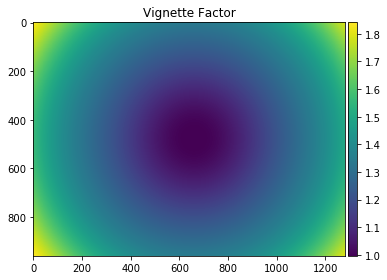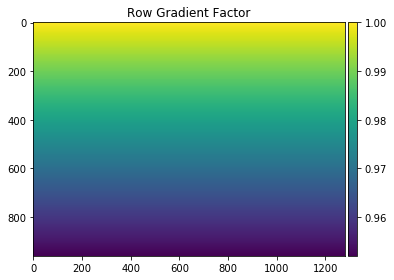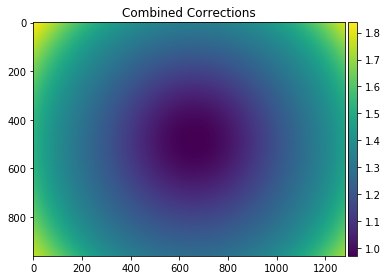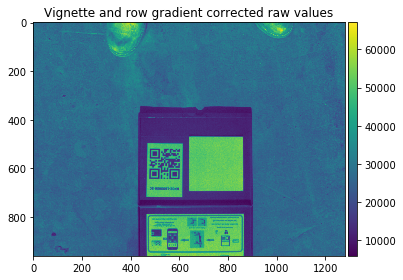# MicaSense RedEdge Image Processing Tutorial 1¶

## Overview¶

This tutorial assumes you have gone through the basic setup here and your system is set up and ready to go.

In this tutorial, we will walk through how to convert RedEdge data from raw images to radiace and then to reflectance. We will cover the tools required to do this, and walk through some of the basic image processing and radiometric conversions.

### Opening an image with pyplot¶

RedEdge 16-bit images can be read with pyplot directly into numpy arrays using the pyplot imread function or the matplotlib imread function, and then we can display the image inline using the imshow function of matplotlib.

In :
import cv2
import matplotlib.pyplot as plt
import numpy as np
import os,glob
import math
%matplotlib inline

imagePath = os.path.join('.','data','0000SET','000')
imageName = os.path.join(imagePath,'IMG_0000_4.tif')

# Read raw image DN values
# reads 16 bit tif - this will likely not work for 12 bit images

# Display the image
fig, ax = plt.subplots(figsize=(8,6))
ax.imshow(imageRaw, cmap='gray')
plt.show()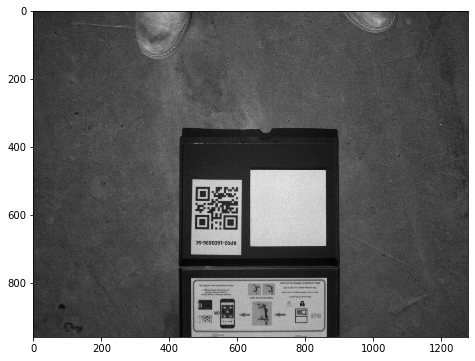### MicaSense Utilities Module¶

For many of the steps in the tutorial, we will use code from the MicaSense utilities module. The code is in the micasense directory and can be imported via normal python import commands using the syntax import micasense or import micasense.submodule as short_name for use in this and other scripts. While we will not cover all of the utility functions in this tutorial, they are available for reference and some will be used and discussed in future tutorials.

We will use start by using a plotting function in micasense.plotutils that adds a colorbar to the display, so that we can more easily see changes in the values in the images and also see the range of the image values after various conversions. This function also colorizes the grayscale images, so that changes can more easily be seen. Depending on your viewing style, you may prefer a different color map and you can also select that colormap here or browsing the colormaps on the matplotlib site.

In :
import micasense.plotutils as plotutils

# Optional: pick a color map that fits your viewing style
# one of 'gray, viridis, plasma, inferno, magma, nipy_spectral'
plotutils.colormap('viridis');

fig = plotutils.plotwithcolorbar(imageRaw, title='Raw image values with colorbar')

<Figure size 432x288 with 0 Axes>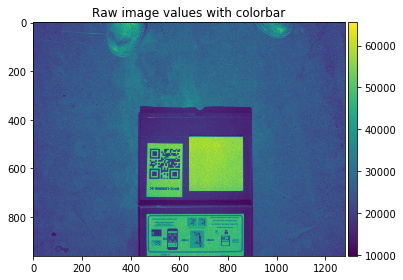In order to perform various processing on the images, we need to read the metadata of each image. For this we use ExifTool. We can read standard image capture metadata such as location, UTC time, imager exposure and gain, but also RedEdge specific metadata which can make processing workflows easier.

For example, each image contains a unique capture identifier. Capture identifiers are shared between all 5 images captured by RedEdge at the same moment, and can be used to unambiguously group images in post processing, regardless of how the images are named or stored on disk. Each image also contains a flight identifer which is the same for all images taken during a single power cycle of the camera. This can be used in post-processing workflows to group images and in many cases, more easily identify when the vehicle took off and landed.

In :
import micasense.metadata as metadata
exiftoolPath = None
if os.name == 'nt':
exiftoolPath = os.environ.get('exiftoolpath')
cameraMake = meta.get_item('EXIF:Make')
cameraModel = meta.get_item('EXIF:Model')
firmwareVersion = meta.get_item('EXIF:Software')
bandName = meta.get_item('XMP:BandName')
print('{0} {1} firmware version: {2}'.format(cameraMake,
cameraModel,
firmwareVersion))
print('Exposure Time: {0} seconds'.format(meta.get_item('EXIF:ExposureTime')))
print('Imager Gain: {0}'.format(meta.get_item('EXIF:ISOSpeed')/100.0))
print('Size: {0}x{1} pixels'.format(meta.get_item('EXIF:ImageWidth'),meta.get_item('EXIF:ImageHeight')))
print('Band Name: {0}'.format(bandName))
print('Center Wavelength: {0} nm'.format(meta.get_item('XMP:CentralWavelength')))
print('Bandwidth: {0} nm'.format(meta.get_item('XMP:WavelengthFWHM')))
print('Capture ID: {0}'.format(meta.get_item('XMP:CaptureId')))
print('Flight ID: {0}'.format(meta.get_item('XMP:FlightId')))
print('Focal Length: {0}'.format(meta.get_item('XMP:FocalLength')))

MicaSense RedEdge firmware version: v2.1.2-34-g05e37eb-local
Exposure Time: 0.0018 seconds
Imager Gain: 1.0
Size: 1280x960 pixels
Band Name: NIR
Center Wavelength: 840 nm
Bandwidth: 40 nm
Capture ID: 5v25BtsZg3BQBhVH7Iaz
Flight ID: NtLNbVIdowuCaWYbg3ck
Focal Length: None


### Converting raw images to Radiance¶

Ultimately most RedEdge users want to calibrate raw images from the camera into reflectance maps. This can be done using off-the-shelf software from third parties, but you are here because there is no fun in that! Along with this tutorial we have included some helper utilities that will handle much of this conversion for you, but here we will walk through a few of those functions to discuss what is happening inside.

Any RedEdge workflow must include these common steps.

1. Un-bias images by accounting for the dark pixel offset
2. Compensate for imager-level effects
3. Compensate for optical chain effects
4. Normalize images by exposure and gain settings
5. Convert to a common unit system (radiance)

All of these are handled by the micasense.utils.raw_image_to_radiance(metadata, raw_image) function. Let us take a look at that fuction in more detail.

First, we get the darkPixel values. These values come from optically-covered pixels on the imager which are exposed at the same time as the image pixels. They measure the small amount of random charge generation in each pixel, independent of incoming light, which is common to all semiconductor imaging devices.

blackLevel = np.array(meta.get_item('Exif.BlackLevel'))
darkLevel = blackLevel.mean()


Now, we get the imager-specific calibrations.

a1, a2, a3 = meta.get_item('XMP:RadiometricCalibration')


We get the parameters of the optical chain (vignette) effects and create a vignette map. This map will be multiplied by the black-level corrected image values to reverse the darkening seen at the image corners. See the vignette_map function for the details of the vignette parameters and their use.

V, x, y = vignette_map(meta, xDim, yDim)


Now we can calculate the imager-specfic radiometric correction function, which help to account for the radiometric inaccuracies of the CMOS imager pixels.

# row gradient correction
R = 1.0 / (1.0 + a2 * y / exposureTime - a3 * y)


Finally, we apply these functions to the raw image to result in a corrected image

# subtract the dark level and adjust for vignette and row gradient
L = V * R * (imageRaw - darkLevel)


Next, we get the exposure and gain settings (gain is represented in the photographic parameter ISO, with a base ISO of 100, so we divide the result to get a numeric gain).

exposureTime = float(meta.get_item('EXIF:ExposureTime'))
gain = float(meta.get_item('EXIF:ISOSpeed'))/100.0


Now that we have a corrected image, we can apply a conversion from calibrated digital number values to radiance units (W/m^2/nm/sr). Note that in this conversion, we need to normalize by the image bitdepth (2^16 for 16 bit images, 2^12 for 12-bit images), because the calibration coefficients are scaled to work with normalized input values.

# apply the radiometric calibration -
# scale by the gain-exposure product and multiply with the radiometric calibration coefficient
bitsPerPixel = meta.get_item('EXIF:BitsPerSample')
dnMax = float(2**bitsPerPixel)


For convenience, we have written the raw_image_to_radiance function to return the intermediate compensation images as well, so we can visualize them for the tutorial. These intermediate results are not required in most implementations and can be ommitted, if performance is a concern.

In :
import micasense.utils as msutils